# I.E. Irodov Solutions on Equation of the Gas State Processes

Students can find the solutions of the Problems on Equation of the Gas State Processes covered in I.E. Irodov book on this page. All the solutions are solved in detail by BYJU'S subject matter experts. I.E. Irodov Solutions for PART TWO - THERMODYNAMICS AND MOLECULAR PHYSICS - 2.1. Equation of the Gas State Processes are provided here to help the students in their effective preparation for the JEE Main and other competitive exams. Students can now solve any problem from the I.E. Irodov book by referring to the I.E. Irodov Solutions.

### I.E. Irodov Solutions on Equation of the Gas State Processes

1. A vessel of volume V = 30 l contains ideal gas at the temperature 0°C. After a portion of the gas has been let out, the pressure in the vessel decreased by ∆p = 0.78 atm (the temperature remaining constant). Find the mass of the released gas. The gas density under the normal conditions ρ = 1.3 g/l.

Solution:

1. Let m1 and m2 be the masses of the gas in the vessel before and after the gas is released.

Mass of the gas released, ∆m = m1 - m2

Now from idea gas equation: p1V = m1(R/M)To and p2V = m2(R/M)To

as V and T are same before and after the release of the gas.

So, (p1 - p2)V = (m1 – m2)(R/M)To = ∆m(R/M)To

Or ∆m = $\frac{(p_1-p_2)VM}{RT_o}$

= ∆pVM/RTo …(1)

We know, p = ρ(R/M)T . so, M/RTo = ρ/po ….(2)

From (1) and (2), we have

∆m = ρV(∆p/po) = 1.3 x 30 x 0.78/1 = 30g

2. Two identical vessels are connected by a tube with a valve letting the gas pass from one vessel into the other if the pressure difference ∆p ≥ 1.10 atm. Initially there was a vacuum in one vessel while the other contained ideal gas at a temperature t1 = 27°C and pressure p1 = 1.00 atm. Then both vessels were heated to a temperature t2 =107°C. Up to what value will the pressure in the first vessel (which had vacuum initially) increase?

Solution:

1. Let m1 be the mass of the gas enclosed.

Then, p1V = γ1RT1

When heated, some gas, passes into the evacuated vessel till pressure difference becomes ∆p. Let p1' and p2' be the pressure on the two sides of the valve.

Then, p1' V = γ1’RT2 and p2'V = γ2’RT2 = (γ1 – γ1’)RT2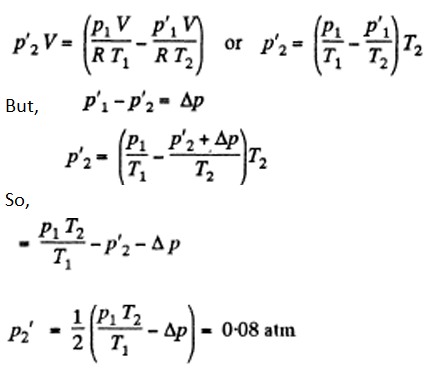3. A vessel of volume V = 20 l contains a mixture of hydrogen and helium at a temperature t = 20°C and pressure p = 2.0 atm. The mass of the mixture is equal to m = 5.0 g. Find the ratio of the mass of hydrogen to that of helium in the given mixture.

Solution:

1. Let the mixture contain v1 and v2 moles of H2 and He respectively. If molecular weights of H2 and He are M1 and M2, then respective masses in the mixture are equal to

m1 = v1M1 and m2 = v2M2

Therefore, for the total mass of the mixture is m = m1 + m2 or m = v1 M1 + v2 M2 .....(1)

Also, if v is the total number of moles of the mixture in the vessels, then we know,

V = V1 + V2 ...(2)

Solving (1) and (2), we have

$v_1 = \frac{vM_2-m}{M_2 - M_1}, \ v_2 = \frac{m - vM_1}{M_2 - M_1}$

$m_1 = M_1\frac{vM_2-m}{M_2 - M_1}, \ m_2 = M_2\frac{m - vM_1}{M_2 - M_1}$

or $\frac{m_1}{m_2} = (\frac{M_1}{M_2}) (\frac{vM_2-m}{m-vM_1})$

Above result can be expressed in terms of the effective molecular weight M of the mixture as,

M = m/v = m(RT/pV)

$\frac{m_1}{m_2} = \frac{M_1}{M_2}.\frac{M_2-M}{M - M_1} = \frac{1-M/M2}{M/M_1-1}$

Using given values, we get

M = 3.0g and m1/m2 = 0.50

4. A vessel contains a mixture of nitrogen (m1 = 7.0 g) and carbon dioxide (m2 = 11 g) at a temperature T = 290 K and pressure p0, = 1.0 atm. Find the density of this mixture, assuming the gases to be ideal.

Solution:

1. We know, for the mixture, N2 and CO2 (being regarded as ideal gases, their mixture too behaves like an ideal gas)

pV = vRT so poV = vRT

Where, v = total number of moles of the gases (mixture) present

V = Volume of the vessel.

If v1 and v2 are number of moles of N2 and CO2 respectively present in the mixture, then

v = v1 + v2

The number of moles of N2 and CO2 is,

v1 = m1/M1 and v2 = m2/M2

Here, m1 = mass of N2 (Molecular weight = M1) in the mixture

m2 = mass of CO2 (Molecular weight = M2) in the mixture.

Therefore, density of the mixture is given by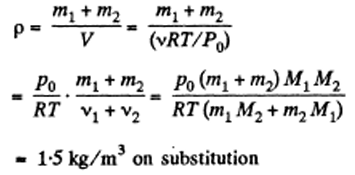5. A vessel of volume V = 7.5 l contains a mixture of ideal gases at a temperature T = 300 K: v1 = 0.10 mole of oxygen, v2 = 0.20 mole of nitrogen, and v3 = 0.30 mole of carbon dioxide. Assuming the gases to be ideal, find:

1. (a) the pressure of the mixture;

2. (b) the mean molar mass M of the given mixture which enters its equation of state pV = (m/M) RT, where m is the mass of the mixture.

Solution:

1. (a) The mixture contains v1, v2 and v3 moles of O2 > N2 and CO2 respectively. Then the total number of moles of the mixture is

v = v1 + v2 + v3 ...(1)

We know, ideal gas equation for the mixture is

pV = vRT or p = (vRT)/V

p = [(v1 + v2 + v3)RT]/V (Using (1)

Using given data, we get

p = 1.968 atm

(b) M ass of oxygen (O2) present in the mixture: m1 = v1 M1

Mass of nitrogen (N2) present in the mixture: m2 = v2M2

Mass of carbon dioxide (CO2) present in the mixture: m3 = v3M3

So, mass of the mixture

m = m1 + m2 + m3 = v1 M1 + v2 M2 + v3 M3

If M is the molecular mass of the mixture, then M = (mass of the mixture)/(total number of moles)

Or M = (v1 M1 + v2 M2 + v3 M3)/( v1 + v2 + v3)

Or M = 36.7 g/mol

6. A vertical cylinder closed from both ends is equipped with an easily moving piston dividing the volume into two parts, each containing one mole of air. In equilibrium at T0 = 300 K the volume of the upper part is η = 4.0 times greater than that of the lower part. At what temperature will the ratio of these volumes be equal η' = 3.0?

Solution:

1. Let p1 and p2 be the pressure in the upper and lower part of the cylinder respectively at temperature T0. At the equilibrium position for the piston:

Let m is the mass of the piston.

p1S + mg = p2S or p1 + mg/S = p2

But p1 = RTo/ ηVo

where V0 is the initial volume of the lower part.

So, RTo/ ηVo + mg/S = RTo/Vo or mg/S = (RTo/Vo )(1 – 1/η) …(1)

Let T be the sought temperature and at this temperature the volume of the lower part becomes V, then according to the problem the volume of the upper part becomes η'V'.

mg/S = (RT/V’ )(1 – 1/η’) …(2)

From (1) and (2), we have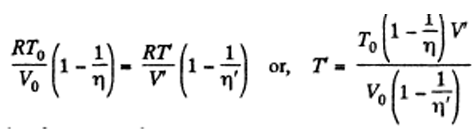…(3)

Total volume must be constant

Vo(1 + η) = V’(1 + η’) or V’ = [Vo(1 + η)]/ (1 + η’)

Put the value of V in equation (3)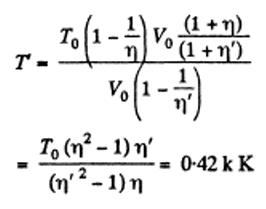7. A vessel of volume V is evacuated by means of a piston air pump. One piston stroke captures the volume ΔV. How many strokes are needed to reduce the pressure in the vessel η times? The process is assumed to be isothermal, and the gas ideal.

Solution:

1. Let P1 be the density after the first stroke. The mass remains constant V ρ = (V + ΔV) ρ1 …(1)

Similarly, if p2 is the density after second stroke

V ρ1 = (V + ΔV) ρ2

Using (1), we have

ρ2 = (V/(V + ΔV))2 ρ0

In this way after nth stroke.

ρn = (V/(V + ΔV))n ρ0

Since pressure α density,

pn = (V/(V + ΔV))n p0

(as temperature is constant)

It is required by pn/po to be 1/η

So, 1/η = ( V/(V + ΔV))n

Hence, n = (ln η )/(ln(1 + ΔV/V))

8. Find the pressure of air in a vessel being evacuated as a function of evacuation time t. The vessel volume is V, the initial pressure is P0. The process is assumed to be isothermal, and the evacuation rate equal to C and independent of pressure.

Note: The evacuation rate is the gas volume being evacuated per unit time, with that volume being measured under the gas pressure attained by that moment.

Solution:

1. From the ideal gas equation: p = (m/M)(RT/V)

dp/dt = RT/MV x dm/dt ……(1)

In each stroke, volume v of the gas is ejected, where v is given by

$v = \frac{V}{m_N}[m_{N-1}-m_N]$

In case of continuous ejection, if (mN-1) corresponds to mass of gas in the vessel at time t, then mN is the mass at time t + Δt, where Δt, is the time in which volume v of the gas has come out. The rate of evacuation is therefore

C = v/ Δt = $\frac{-V}{m(t+ \Delta t)}. \frac{m(t+ \Delta t)- m(t)}{ \Delta t }$

In the limit Δt ->o, we have

C = V/m x dm/dt …(2)

From (1) and (2)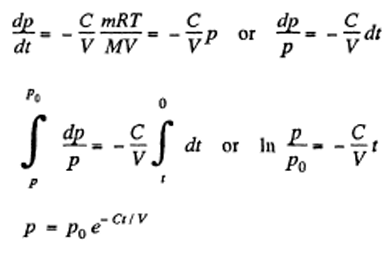9. A chamber of volume V = 87 l is evacuated by a pump whose evacuation rate (see Note to the foregoing problem) equals C = 10 1/s. How soon will the pressure in the chamber decrease by η = 1000 times?

Solution:

1. Let p be the instantaneous density, then instantaneous mass = Vp.

In a short, interval dt the volume is increased by C dt.

So, Vρ = (V + C dt)( ρ + d ρ)

(as mass remains constant in a short interval dt)

Dρ/ρ = -C/V dt

Since pressure α density dp/P = -C/V dt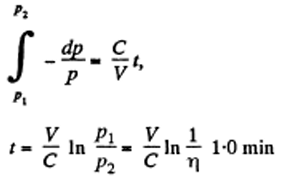10. A smooth vertical tube having two different sections is open from both ends and equipped with two pistons of different areas (fig given below). Each piston slides within a respective tube section. One mole of ideal gas is enclosed between the pistons tied with a non-stretchable thread. The cross-sectional area of the upper piston is ΔS = 10 cm2 greater than that of the lower one. The combined mass of the two pistons is equal to m = 5.0 kg. The outside air pressure is P0 = 1.0 atm. By how many kelvins must the gas between the pistons be heated to shift the pistons through l = 5.0 cm?Solution:

1. The physical system consists of one mole of gas confined in the smooth vertical tube. Let m1 and m2 be the masses of upper and lower pistons and S1 and S2 are their respective areas.

For the lower piston:

pS2 + m2g = p0S2 + T

or T = (p – p0)S2 + m2g ….(1)

For the upper piston:

pS1 + T + m1g = pS1

or T = (p – p0)S1 – m1g ….(2)

From (1) and (2)

(p – p0)(S1 – S2) = (m1 +m2)g

Or (p – p0) ∆S = mg

So, p = mg/∆S + p0 = constant

From gas law, pV = vRT

p∆V = vR∆T

(p is constant here)

So, (p0 + mg/∆S) ∆S l =R∆T

Hence, ∆T = (1/R) (p0 ∆S + mg) l = 0.9 k

11. Find the maximum attainable temperature of ideal gas in each of the following processes:

(a) p = po – αV2

(b) p = po e- βV

Where p0, α and β are positive constants, and V is the volume of one mole of gas.

Solution:

1. (a) p = po – αV2 = po – α(RT/p)2

We know, V = RT/p for one mole of gas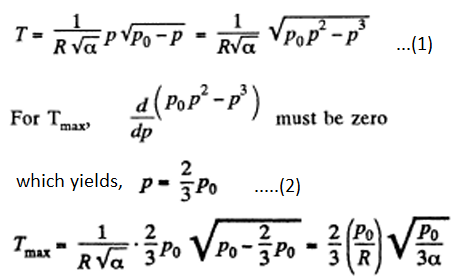(b) p = po e- βV = po e- βRT/p

βRT/p = ln po/p and T = p/βR) ln(po/p) …(1)

For Tmax the condition is dT/dp = 0, which yields p = P0/e

Using above value of o in equation (1), we get

Tmax = po/eβR

12. Find the minimum attainable pressure of ideal gas in the process T = T0 + αV2, where T0 and α are positive constants, and V is the volume of one mole of gas.

Solution:

1. T = T0 + αV2 = T0 + α(RT/p)2

P = √α RT(T – To)1/2 …(1)

For Pmin, dp/dT = o

T = 2To …(2)

From (1) and (2), we get,

pmin = √α R(2To)(2To – To)-1/2 = 2R√(αTo)

13. A tall cylindrical vessel with gaseous nitrogen is located in a uniform gravitational field in which the free-fall acceleration is equal to g. The temperature of the nitrogen varies along the height h so that its density is the same throughout the volume. Find the temperature gradient dT/dh.

Solution:

1. Consider a thin layer at a height h and thickness dh. Let p and dp+p be the pressure on the two sides of the layer. The mass of the layer is Sdhp. Equating vertical downward force to the upward force acting on the layer.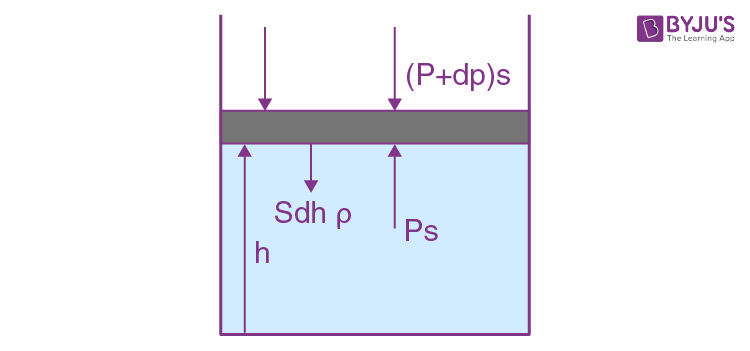dp/dh = -ρg ...(1)

But p = (ρ/M) RT

dp = (ρR/M)dT

or (-ρR/M)dT = ρ g dh

so, dT/dh = (-gM/R) = -34 K/km

which shows that, temperature of air drops by 34°C at a height of 1 km above bottom.

14. Suppose the pressure p and the density p of air are related as p/pn = const regardless of height (n is a constant here). Find the corresponding temperature gradient.

Solution:

1. As, dp/dh = - ρg

But, p = C ρn

Where C is constant

dp/dρ = Cn ρn-1 ……(2)

From gas law, P = ρT(R/M) [using (2)]

n = ρT(R/M) or T = (M/R) Cρn-1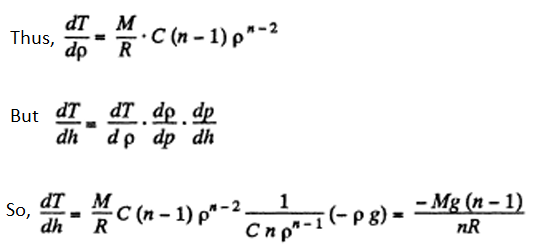15. Let us assume that air is under standard conditions close to the Earth's surface. Presuming that the temperature and the molar mass of air are independent of height, find the air pressure at the height 5.0 km over the surface and in a mine at the depth 5.0 km below the surface.

Solution:

1. We have, dp = - p g dh and from gas law ρ = (M/RT)p

dp/P = (-Mg/RT)dh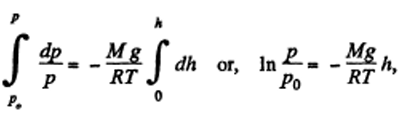Where p0 is the pressure at the surface of the Earth.

p = p0e-Mgh/RT

At po = 1 atm and T = 273 K

Pressure at a height of 5 atm = 1 x e-28x9.81x5000/8314x273 = 0.5 atm

Pressure in a mine at a depth of 5 km = 1 x e-28x9.81x-5000/8314x273 = 2 atm

16. Assuming the temperature and the molar mass of air, as well as the free-fall acceleration, to be independent of the height, find the difference in heights at which the air densities at the temperature 0°C differ

(a) e times; (b) by η = 1.0%.

Solution:

1. We know, dp = - pg dh but from gas law p= (ρ /M)RT

Thus, dp = (d ρ/M)RT at const, temperature

So, dρ/ ρ = (gM/RT)dh

Integrating above equation, we have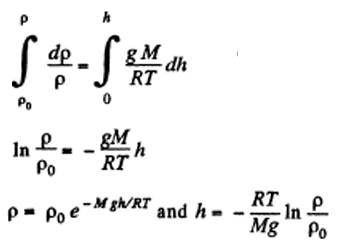(a) ρo/ ρ = e and T = 273K

Thus, h = (-RT/Mg) ln e-1 = 8 km

(b) (ρo – ρ)/ ρo = 0.01 or ρ/ρo = 0.99

Thus, h = -(RT/Mg) ln ρ/ρo = 0.99

17. An ideal gas of molar mass M is contained in a tall vertical cylindrical vessel whose base area is S and height h. The temperature of the gas is T, its pressure on the bottom base is P0. Assuming the temperature and the free-fall acceleration g to be independent of the height, find the mass of gas in the vessel.

Solution:

1. From the Barometric formula, we have

p = p0e-Mgh/RT and from gas law ρ = pM/RT

at constant temperature,

ρ = (poM/RT) e-Mgh/RT = ρ0e-Mgh/RT …(1)

Here equation (1) shows that density varies with height in the same manner as pressure. Let us consider the mass element of the gas contained in the contemn.

dm = ρ(Sdh) = ( Mpo/RT) e-Mgh/RT Sdh

The sought mass is,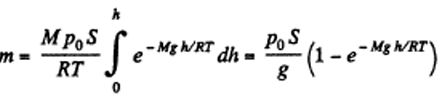18. An ideal gas of molar mass M is contained in a very tall vertical cylindrical vessel in the uniform gravitational field in which the free-fall acceleration equals g. Assuming the gas temperature to be the same and equal to T, find the height at which the centre of gravity of the gas is located.

Solution:

1. As the gravitational field is constant the centre of gravity and the centre of mass are same. The location of C.M.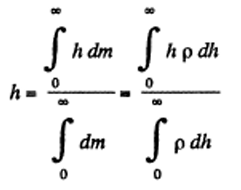But from Barometric formula and gas law ρ = ρ0e-Mgh/RT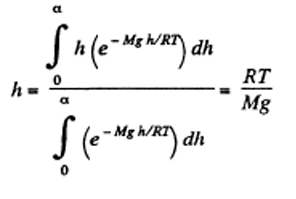19. An ideal gas of molar mass M is located in the uniform gravitational field in which the free-fall acceleration is equal to g. Find the gas pressure as a function of height h, if p = p0 at h = 0, and the temperature varies with height as (a) T = T0 (1 - ah); (b) T = T0 (1 + ah), where a is a positive constant.

Solution:

1. The variation of pressure with height of a fluid is given by : dp = - p g dh

But from gas law: ρ = pM/RT

So, dp = (-pMg/RT)dh …(1)

Or dp/p = [-Mgdh]/[RT0(1-ah)]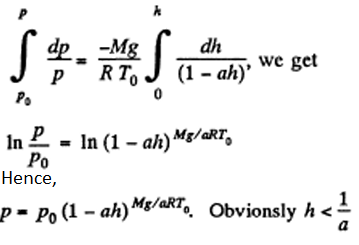(b) Proceed up to equation (1) of part (a), and then substitute T = T0(1 + ah) and proceed further in the same fashion to get

p = p0/(1+ah)Mg/aRT_0

20. A horizontal cylinder closed from one end is rotated with a constant angular velocity ω about a vertical axis passing through the open end of the cylinder. The outside air pressure is equal to P0 , the temperature to T, and the molar mass of air to M. Find the air pressure as a function of the distance r from the rotation axis. The molar mass is assumed to be independent of r.

Solution:

1. Let us consider the mass element of the gas (thin layer) in the cylinder at a distance r from its open end as shown in the figure.

Using Newton’s second law for the element So,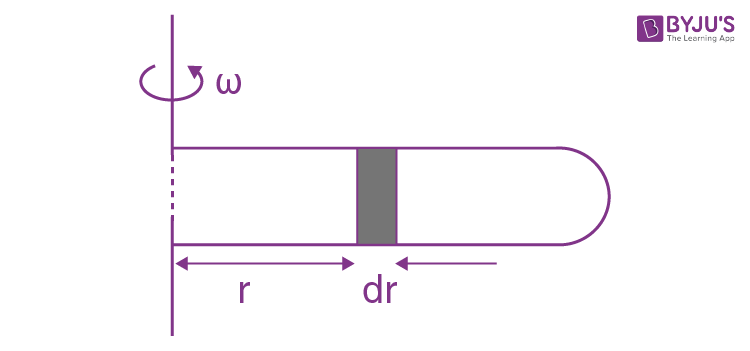21. Under what pressure will carbon dioxide have the density p = 500 g/l at the temperature T = 300 K? Carry out the calculations both for an ideal and for a Van der Waals gas.

Solution:

1. For an ideal gas law, p = (ρ/M)RT

So, p = 0.082 x 300 x 500/44 atm = 279.5 atm

For Vander Waal gas Equation,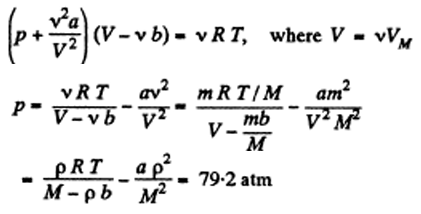22. One mole of nitrogen is contained in a vessel of volume V = 1.00 l. Find: (a) the temperature of the nitrogen at which the pressure can be calculated from an ideal gas law with an error η = 10% (as compared with the pressure calculated from the Van der Waals equation of state); (b) the gas pressure at this temperature.

Solution:

1. (a) The pressure is less for a Vander Waal gas than for an ideal gas) Or Or , , (b) The corresponding pressure is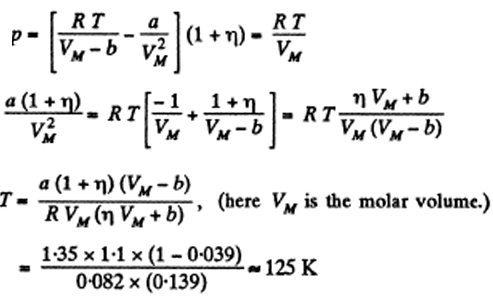(b) The corresponding pressure is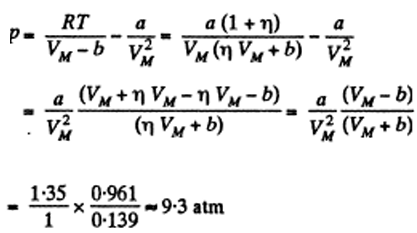23. One mole of a certain gas is contained in a vessel of volume V = 0.250 l. At a temperature T1 = 300 K the gas pressure is p1 = 90 atm, and at a temperature T2 = 350 K the pressure is p2 = 110 atm. Find the Van der Waals parameters for this gas.

Solution:

1. $p_1 = RT_1 \frac{1}{V-b} - \frac{a}{V^2}, \ p_2= RT_2 \frac{1}{V-b} - \frac{a}{V^2}$

$p_2 – p_1 = \frac{R(T_2-T_1)}{V-b}$

Or $V-b = \frac{R(T_2-T_1)}{p_2-p_1}$

Or $b = V - \frac{R(T_2-T_1)}{p_2-p_1}$

Also,

$p_1 = T_1 \frac{ (p_2-p_1)}{T_2-T_1} -\frac{a}{V^2}$

$\frac{a}{V^2} = T_1 \frac{ (p_2-p_1)}{T_2-T_1} -p_1$

Or $a = V^2 \frac{T_1p_2-p_1T_2}{T_2-T_1}$

Using given data, we have

A = 1.87 atm. litre2/mole2 and b = 0.045 litre/mole

24. Find the isothermal compressibility x of a Van der Waals gas as a function of volume V at temperature T.

Note. By definition x = $\frac{-1}{V} \frac{\partial V}{\partial p}$

Solution:

1.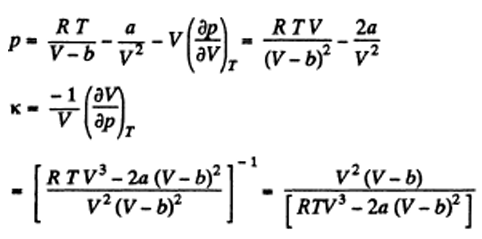25. Making use of the result obtained in the foregoing problem, find at what temperature the isothermal compressibility x of a Van der Waals gas is greater than that of an ideal gas. Examine the case when the molar volume is much greater than the parameter b.

Solution:

1. For an ideal gas: ko = V/RT

$\kappa = \frac{(V-b)^2}{RTV}[1 - \frac{2a(V-b)^2}{RTV^3}]^{-1}$

$= \kappa_0(1 - \frac{b}{V})^2 [1 - \frac{2a}{RTV}(1 - \frac{b}{V})^2]^{-1}$

$= \kappa_0(1 - \frac{2b}{V} + \frac{2a}{RTV})$, to leading order in a, b

Now, $\kappa > \kappa_0\ if\ \frac{2a}{RTV} > \frac{2b}{V}$

Or T < a/bR

If a, b do not vary much with temperature, then the effect at high temperature is clearly determined by b and its effect is repulsive so compressibility is less